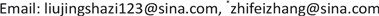1佛山科学技术学院自动化学院，广东 佛山

2广东立胜综合能源服务有限公司，广东 佛山1. 引言

2. 混合集成模型2.1. 样本数据的预处理

y i ⇒ ∑ j = 1 q β i j x i j + ∑ j 1 = 1 q ∑ j 2 = 1 q γ i j 1 j 2 x i j 1 x i j 2 。 (1)

PCA方法根据阀值可直接确定出主影响因素，非线性回归则是检测各影响因素的置信度来决定主要影响变量，具体的操作方法见文末实例分析。

2.2. 混合集成原理

H k = ∑ i = 1 T k α k i g k i ， (2)

S = f ( H 1 , H 2 , ⋯ , H n ) ， (3)

3. 分类器算法

For k = 1 to n，

For t = 1 to ，

1) 输入数据 X = { ( x 1 , y 1 ) , ( x 2 , y 2 ) , ⋯ , ( x m , y m ) } ，样本的初始分布权值 D t ( i ) = 1 / m 。

2) 弱分类器预测。

e t = ∑ i = 1 m D t ( i ) | g ( i ) − y ( i ) | ， i = 1 , 2 , 3 , ⋯ , m ， (4)

3) 根据弱分类器预测误差et计算弱分类器权重αt

α t = 1 2 ln ( 1 − e t e t ) 。 (5)

4) 更新样本的分布权值，调整公式为：

IF (模型输出 ≠ 期望输出) and (期望输出为患病类别)

D ( t + 1 ) ( i ) = k 1 D t ( i ) exp [ − α t y i g t ( x i ) ] ， i = 1 , 2 , ⋯ , m ， k 1 ≥ 1 。 (6)

IF (模型输出 ≠ 期望输出) and (期望输出为健康类别)

D ( t + 1 ) ( i ) = k 2 D t ( i ) exp [ − α t y i g t ( x i ) ] ， i = 1 , 2 , ⋯ , m ， 0 < k 2 < 1 。 (7)

ELSE D ( i + 1 ) = D i 。 (8)

D s u m = s u m ( D t + 1 ( i ) ) (9)

D t + 1 ( i ) = D t + 1 ( i ) / D s u m 。 (10)

Next t；

5) 得到由 T k 组弱分类函数组合得到第k种算法的强分类函数 H k ( x ) ：

H k ( x ) = s i g n [ ∑ t = 1 T α t h T k ( x ) ] (11)

Next k。

4. 实验及分析4.1. 数据预处理

Dataset propertie

1半径(中心到圆周上各点距离的平均值)6紧密度
2纹理(灰度值的标准差)7凹陷度(轮廓凹部的严重程度)
3周长8凹陷点数(轮廓凹面部分的数量)
4面积9对称度
5平滑度(半径长度的局部变化)10断裂度

4.1.1. 主分分析

PCA是运用最广泛的线性降维方法之一，主成分分析的实质是：通过正交变换将数据转换为相等数量的线性不相关变量，尽可能保留原始数据特征。PCA算法的主要步骤如下：

1) 输入样本数据 X = { X 1 , X 2 , ⋯ , X n } 为n行m列，对数据进行标准化得到矩阵M，

M = X i j − X ¯ j v a r ( X j ) ,     i = 1 , 2 , ⋯ , n ;   j = 1 , 2 , ⋯ , m ， (12)

X ¯ j = 1 n ∑ i = 1 n X i j , v a r ( X j ) = 1 n − 1 ∑ i = 1 n ( X i j − X ¯ j ) 2 ,     j = 1 , 2 , ⋯ , m 。 (13)

2) 求矩阵M对应的协方差矩阵：

M b = 1 n − 1 M T M 。 (14)

3) 求矩阵Mb的非负的特征根 λ 1 > λ 2 > ⋯ > λ P ≥ 0 ，p为非负特征根的数量， λ i 对应的特征向量记为：

v i = ( v i 1 , v i 2 , ⋯ , v i P ) , i = 1 , 2 , ⋯ , P 。 (15)

v i v j T = ∑ k = 1 P v i k v j k = { 1 i = j 0 i ≠ j 。 (16)

4) 计算累计贡献率即某个特征值占全部特征值合计的比重：

η = ∑ λ i ∑ i = 1 P λ i 。 (17)

4.1.2. 逐步回归分析

4.2. 评价指标

a) 准确性：相对于测试的样本总数sum，正确分类为给定类别的肿瘤的百分比，公式为：

Accury = TP + TN sum 。 (18)

b) 漏诊率MDR (Missed diagnosis rate)：漏诊率是实际为恶性肿瘤的样本中，预测为良性的占比，公式为：

MDR = TP TP + FP 。 (19)

c) 灵敏度Sen (Sensitivity)：研究对象诊断为恶性肿瘤的概率，公式为：

Sen = TP TP + FN 。 (20)

d) 特异度Spe (Specificity)：实际上良性被诊断为良性的概率，公式为：

S e n = T P T P + F N 。 (21)

e) 约登指数：是评价筛查试验真实性的方法，公式为：

Y o u d e n = T P T P + F N + T N T N + F P − 1 。 (22)

f) 误差率：相对于测试的总样本数sum，错误分类为给定类别的肿瘤的百分比，公式为：

E r r o r = F P + F N s u m 。 (23)

Stepwise regression and principal component analysi

4.3. 混合集成与单一算法比较

5. 结论